# Timeseries modelling

## Autoregressive (AR)

### Idea

Output variable depends linearly on its own previous values and on a stochastic term (accounting for the variance in the data).

### Model

AR(p) refers to autoregressive model of order p, and is written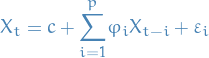1

where

•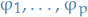are parameters
•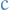is a constant
•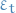is white noise (Gaussian rv.), i.e.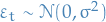The unknowns are:

• constant• the weights / dependence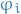of the previous observations
• the variance of the white noise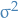### Estimating the parameters

• Ordinary Least Squares (OLS)
• Method of moments (through Yule-Walker equations)

There is a direct correspondance between the parametersand the covariance of the process, and this can be "inverted" to determine the parameters from the autocorrelation function itself.

#### Yule-Walker equations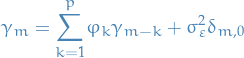2

where

•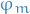is the autocovariance function of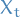•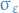is the std. dev. of the input noise process
•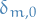is the Kroenecker delta function, which is only non-zero when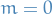Now, if we only consider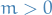, we can ignore the last term, and can simply write the above in matrix form:

#### My thinking

Our plan is as follows:

1. Consider each sequence of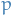steps (the order of the AR)
2. Deduce distribution forconditioned on the parameters of the model and theprevious observations,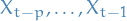.
3. Obtain conditional log-likelihood function for this conditional distribution
4. Get gradient of said conditional log-likelihood function
5. Maximize said conditional log-likelihood wrt. parameters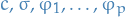6. ???
7. PROFIT!!!
##### Initial observation sequence

We start by collecting the firstobservations in a the sample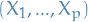, writing it as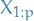, which as a mean vectorwhere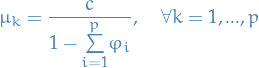3

Why? Well, we start out with the taking the expectation of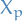wrt. the random variables, i.e.: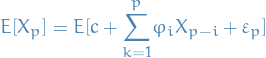4

Then we start expanding: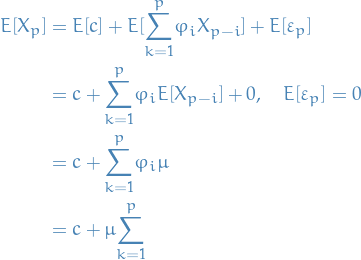5

And since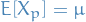, we rearrange to end up with: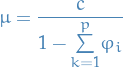6

Voilá!

and the variance-covariance matrix is given by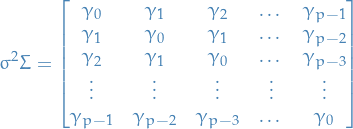7

where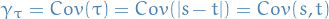for two different stepsand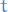. This is due to the process being weak-sense stationary.

The reason for the covariance matrix, is that by our assumption of weak-sense stationarity (is that a word? atleast it is now).

##### Conditional distribution

First we note the following: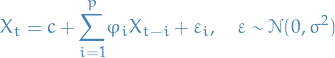8

is equivalent of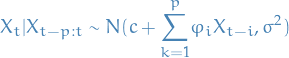9

We then observe that if we conditionon the previousobservations, it is independent on everything before that. That is;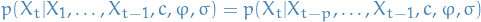10

where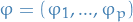.

Therefore,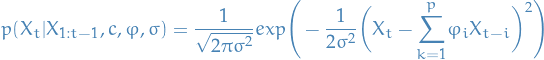11

For notational sake, we note that we can write it in the following form: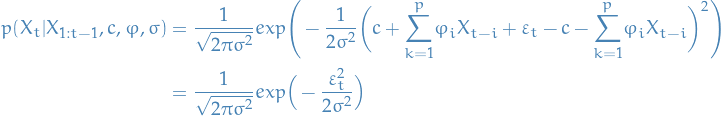12

We can write the total probability of seeing the data conditioned on the parameters as follows: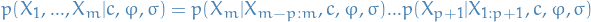13

Which, when we substitute in the normal distributions obtained for,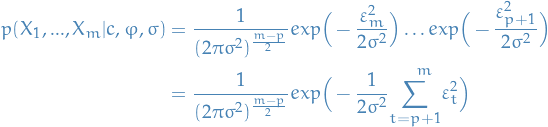14
##### Conditional log-likelihood

Taking the logarithm of the previous expression, we obtain the conditional log-likelihood: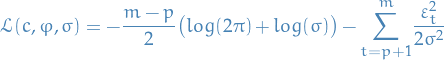15

And just to remind you; what we want to do is find the parameters which maximizes the condition log-likelihood. If we frame it as a minimization problem, simply by taking then egative of the log-likelihood, we end up with the objective function: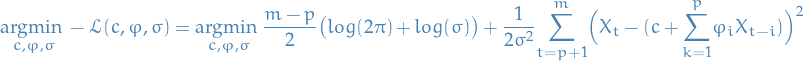16

Now, we optimize!

##### Optimization

If we only consider the objective function wrt. toand, we end up with: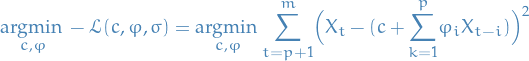17

This is what I arrived at independently, but really thought this was a huge issue becauseis defined as a function of the other variables and so we have a recursive relationship!

But then I realized, after confirming that the above is in fact what you want, that the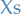in this equation are observed values, not random variables, duh! Might be easier to think about it as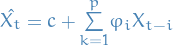, and so we're looking at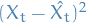, since the last term is in fact our estimate for.

Disclaimer: I think…

Thus, the conditional MLE of these parameters can be obtained from an OLS regression ofon a constantandof its own lagged values.

The conditional MLE estimator ofturns out to be: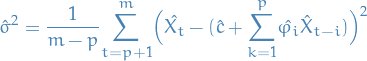18

This can be solved with iterative methods like:

• Gauss-Newton algorithm

Or by finding the exact solution using linear algebra, but the naive approach to this is quite expensive. There are definitively libraries which do this efficiently but nothing that I can implement myself, I think.

## Autoregressive Moving average (ARMA)

Arma(p, q) refers to the model withautoregressive terms and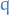moving-average terms. This model contains the AR(p) and MA(q) models,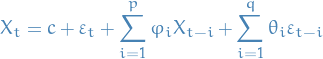## Autoregressive Conditional Heteroskedasticity (ARCH)

### Notation

• Heteroskedastic refers to sub-populations of a collection of rvs. having different variablility

### Stuff

• Describes variance of current error term as a function of the actual sizes of the previous time periods' error terms
• If we assume the error variance is described by an ARMA model, then we have Generalized Autoregressive Conditional Heteroskedasticity (GARCH) model

Letdenote a real-valued discrete-time stochastic process, and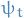and be the information set of all information up to time, i.e.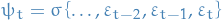.

The process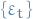is said to be a Autoregressive Conditional Heteroskedasticitic model or ARCH(q), whenever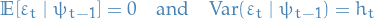with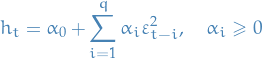The conditional variance can generally be expressed as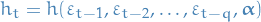where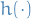is a nonnegative function of its arguments and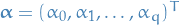is a vector of unknown parameters.

The above is sometimes represented as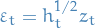with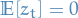and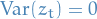, where the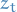are uncorrelated.

We can estimate an ARCH(q) model using OLS.

The Generalized Autoregressive Conditional Heteroskedasticity (GARCH) model or GARCH(p, q) is simply an ARCH(q) in combination with a ARMA(p) model, i.e.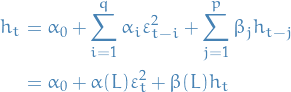where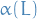and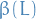are lag operators such that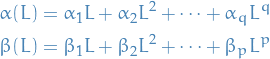Or equivalently,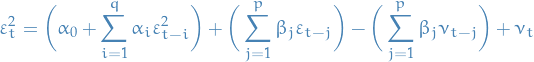where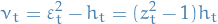which simply ensures that

## Useful tricks

### Autocorrelation using Fourier Transform

Observe that the autocorrelation for a function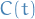can be computed as follows: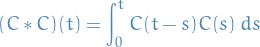since this is just summing over the interactions between every value. If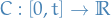, then the above defines the autocorrelation between the series, as wanted.

Now, observe that the [BROKEN LINK: No match for fuzzy expression: def:fourier-transform] has the property: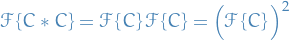Hence,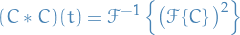Which means that, given a dataset, we can use the efficient Fast Fourier Transform (FFT) to compute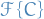, square it, and then take the inverse FFT, to obtain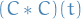, i.e. the autocorrelation!

This is super-dope.

## Appendix A: Vocabulary

autocorrelation
also known as serial correlation, and is the correlation of a signal with a delayed copy of itself as a function of delay. Informally, similarity between observations as a function of the time lag between them.
wide-sense stationary
also known as weak stationary, only require that the mean (1st moment) and autocovariance do not vary wrt. time. This is in contrast to stationary processes, where we require the joint probability to not vary wrt. time.